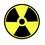# Determinants 4.2

After the 4 by 4 and 5 by 5 matrices of Pascal's triangle, we realise that the determinant of the matrix is 1.

Let $$M_{m,n} = \left( \begin{array}{ccc} m \\ n \\\end{array} \right)$$

Prove that for any matrix of size $a \times a$ in the form $\left( \begin{array}{ccc}M_{0,0} & M_{1,0} & . & . & M_{a,0}\\M_{1,1} & M_{2,1} & & & \\ . & & . & & .\\ . & & & . & .\\ M_{a,a} & . & . & . & M_{2a,a} \end{array} \right)=A$

$det(A)=1$

Or, if you rather...

$det \left( \begin{array}{ccc}\left( \begin{array}{ccc} 0 \\ 0 \\\end{array} \right) & \left( \begin{array}{ccc} 1 \\ 0 \\\end{array} \right) & . & . & \left( \begin{array}{ccc} a \\ 0 \\\end{array} \right) \\\left( \begin{array}{ccc} 1 \\ 1 \\\end{array} \right) & \left( \begin{array}{ccc} 2 \\ 1 \\\end{array} \right) & & & \\ . & & . & & .\\ . & & & . & .\\ \left( \begin{array}{ccc} a \\ a \\\end{array} \right) & . & . & . & \left( \begin{array}{ccc} 2a \\ a \\\end{array} \right) \end{array} \right)=1$

But, there a few methods which may help a lot and will be shared next...Note by Aloysius Ng
6 years, 1 month ago

This discussion board is a place to discuss our Daily Challenges and the math and science related to those challenges. Explanations are more than just a solution — they should explain the steps and thinking strategies that you used to obtain the solution. Comments should further the discussion of math and science.

When posting on Brilliant:

• Use the emojis to react to an explanation, whether you're congratulating a job well done , or just really confused .
• Ask specific questions about the challenge or the steps in somebody's explanation. Well-posed questions can add a lot to the discussion, but posting "I don't understand!" doesn't help anyone.
• Try to contribute something new to the discussion, whether it is an extension, generalization or other idea related to the challenge.

MarkdownAppears as
*italics* or _italics_ italics
**bold** or __bold__ bold
- bulleted- list
• bulleted
• list
1. numbered2. list
1. numbered
2. list
Note: you must add a full line of space before and after lists for them to show up correctly
paragraph 1paragraph 2

paragraph 1

paragraph 2

[example link](https://brilliant.org)example link
> This is a quote
This is a quote
    # I indented these lines
# 4 spaces, and now they show
# up as a code block.

print "hello world"
# I indented these lines
# 4 spaces, and now they show
# up as a code block.

print "hello world"
MathAppears as
Remember to wrap math in $$ ... $$ or $ ... $ to ensure proper formatting.
2 \times 3 $2 \times 3$
2^{34} $2^{34}$
a_{i-1} $a_{i-1}$
\frac{2}{3} $\frac{2}{3}$
\sqrt{2} $\sqrt{2}$
\sum_{i=1}^3 $\sum_{i=1}^3$
\sin \theta $\sin \theta$
\boxed{123} $\boxed{123}$